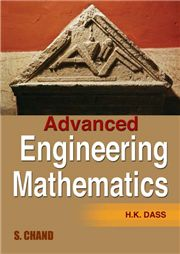# ADVANCED ENGINEERING MATHEMATICS, H K Dass-S.CHAND

825.00 742.00

Partial Differentiation • Multiple Integral • Differential Equations • Determinants and Matrices • Vectors • Complex Numbers • Functions of a Complex Variable • Special Functions • Partial Differential Equations • Statistics • Probability • Fourier Series • Laplace Transformation • Integral Transforms • Numerical Techniques • Numerical Method for Solution of Partial Differential Equation • Calculus of Variations • Tensor Analysis • Z-transforms • Infinite Series • Gamma, Beta Functions • Chebyshev Polynomials • Fuzzy Sets • Hankel Transform • Hilbert Transform • Empirical Laws and Curve Fitting (Method of Least Squares) • Linear Programming